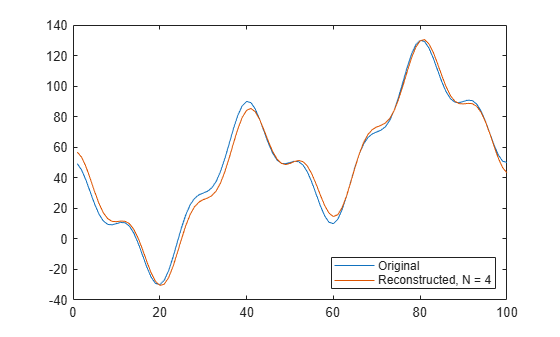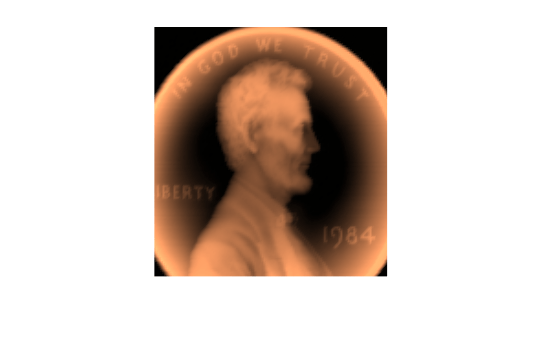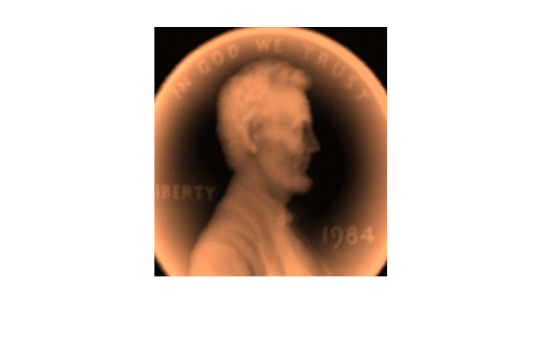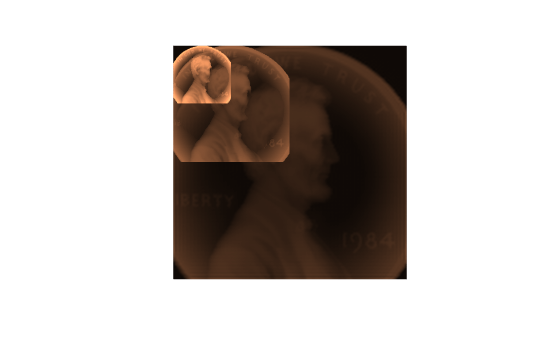# dct

Discrete cosine transform

## Syntax

``y = dct(x)``
``y = dct(x,n)``
``y = dct(x,n,dim)``
``y = dct(___,'Type',dcttype)``

## Description

example

````y = dct(x)` returns the unitary discrete cosine transform of input array `x`. The output `y` has the same size as `x`. If `x` has more than one dimension, then `dct` operates along the first array dimension with size greater than 1.```
````y = dct(x,n)` zero-pads or truncates the relevant dimension of `x` to length `n` before transforming.```

example

````y = dct(x,n,dim)` computes the transform along dimension `dim`. To input a dimension and use the default value of `n`, specify the second argument as empty, `[]`.```

example

````y = dct(___,'Type',dcttype)` specifies the type of discrete cosine transform to compute. See Discrete Cosine Transform for details. This option can be combined with any of the previous syntaxes.```

## Examples

collapse all

Find how many DCT coefficients represent 99% of the energy in a sequence.

```x = (1:100) + 50*cos((1:100)*2*pi/40); X = dct(x); [XX,ind] = sort(abs(X),'descend'); i = 1; while norm(X(ind(1:i)))/norm(X) < 0.99 i = i + 1; end needed = i;```

Reconstruct the signal and compare it to the original signal.

```X(ind(needed+1:end)) = 0; xx = idct(X); plot([x;xx]') legend('Original',['Reconstructed, N = ' int2str(needed)], ... 'Location','SouthEast')```Load a file that contains depth measurements of a mold used to mint a United States penny. The data, taken at the National Institute of Standards and Technology, are sampled on a 128-by-128 grid. Display the data.

```load penny surf(P) view(2) colormap copper shading interp axis ij square off```Compute the discrete cosine transform of the image data. Operate first along the rows and then along the columns.

```Q = dct(P,[],1); R = dct(Q,[],2);```

Find what percentage of DCT coefficients contain 99.98% of the energy in the image.

```X = R(:); [~,ind] = sort(abs(R(:)),'descend'); coeffs = 1; while norm(X(ind(1:coeffs)))/norm(X) < 0.9998 coeffs = coeffs + 1; end fprintf('%3.1f%% of the coefficients are sufficient\n',coeffs/numel(R)*100)```
```21.8% of the coefficients are sufficient ```

Reconstruct the image using only the necessary coefficients.

```R(abs(R) < abs(X(ind(coeffs)))) = 0; S = idct(R,[],2); T = idct(S,[],1);```

Display the reconstructed image.

```surf(T) view(2) shading interp axis ij square off```Load a file that contains depth measurements of a mold used to mint a United States penny. The data, taken at the National Institute of Standards and Technology, are sampled on a 128-by-128 grid. Display the data.

```load penny surf(P) view(2) colormap copper shading interp axis ij square off```Compute the discrete cosine transform of the image data using the DCT-1 variant. Operate first along the rows and then along the columns.

```Q = dct(P,[],1,'Type',1); R = dct(Q,[],2,'Type',1);```

Invert the transform. Truncate the inverse so that each dimension of the reconstructed image is one-half the length of the original.

```S = idct(R,size(P,2)/2,2,'Type',1); T = idct(S,size(P,1)/2,1,'Type',1);```

Invert the transform again. Zero-pad the inverse so that each dimension of the reconstructed image is twice the length of the original.

```U = idct(R,size(P,2)*2,2,'Type',1); V = idct(U,size(P,1)*2,1,'Type',1);```

Display the original and reconstructed images.

```surf(V) view(2) shading interp hold on surf(P) view(2) shading interp surf(T) view(2) shading interp hold off axis ij equal off```## Input Arguments

collapse all

Input array, specified as a real-valued or complex-valued vector, matrix, N-D array, or `gpuArray` object.

See Run MATLAB Functions on a GPU (Parallel Computing Toolbox) and GPU Support by Release (Parallel Computing Toolbox) for details on `gpuArray` (Parallel Computing Toolbox) objects.

Example: `sin(2*pi*(0:255)/4)` specifies a sinusoid as a row vector.

Example: `sin(2*pi*[0.1;0.3]*(0:39))'` specifies a two-channel sinusoid.

Data Types: `single` | `double`
Complex Number Support: Yes

Transform length, specified as a positive integer scalar.

Data Types: `single` | `double`

Dimension to operate along, specified as a positive integer scalar.

Data Types: `single` | `double`

Discrete cosine transform type, specified as a positive integer scalar from 1 to 4. See Discrete Cosine Transform for the definitions of the different types of DCT.

Data Types: `single` | `double`

## Output Arguments

collapse all

Discrete cosine transform, returned as a real-valued or complex-valued vector, matrix, N-D array, or `gpuArray` object.

collapse all

### Discrete Cosine Transform

The discrete cosine transform (DCT) is closely related to the discrete Fourier transform. You can often reconstruct a sequence very accurately from only a few DCT coefficients. This property is useful for applications requiring data reduction.

The DCT has four standard variants. For a signal x of length N, and with δkℓ the Kronecker delta, the transforms are defined by:

• DCT-1:

`$y\left(k\right)=\sqrt{\frac{2}{N-1}}\sum _{n=1}^{N}x\left(n\right)\frac{1}{\sqrt{1+{\delta }_{n1}+{\delta }_{nN}}}\frac{1}{\sqrt{1+{\delta }_{k1}+{\delta }_{kN}}}\mathrm{cos}\left(\frac{\pi }{N-1}\left(n-1\right)\left(k-1\right)\right)$`

• DCT-2:

`$y\left(k\right)=\sqrt{\frac{2}{N}}\sum _{n=1}^{N}x\left(n\right)\frac{1}{\sqrt{1+{\delta }_{k1}}}\mathrm{cos}\left(\frac{\pi }{2N}\left(2n-1\right)\left(k-1\right)\right)$`

• DCT-3:

`$y\left(k\right)=\sqrt{\frac{2}{N}}\sum _{n=1}^{N}x\left(n\right)\frac{1}{\sqrt{1+{\delta }_{n1}}}\mathrm{cos}\left(\frac{\pi }{2N}\left(n-1\right)\left(2k-1\right)\right)$`

• DCT-4:

`$y\left(k\right)=\sqrt{\frac{2}{N}}\sum _{n=1}^{N}x\left(n\right)\mathrm{cos}\left(\frac{\pi }{4N}\left(2n-1\right)\left(2k-1\right)\right)$`

The series are indexed from n = 1 and k = 1 instead of the usual n = 0 and k = 0, because MATLAB® vectors run from 1 to N instead of from 0 to N – 1.

All variants of the DCT are unitary (or, equivalently, orthogonal): To find their inverses, switch k and n in each definition. DCT-1 and DCT-4 are their own inverses. DCT-2 and DCT-3 are inverses of each other.

 Jain, A. K. Fundamentals of Digital Image Processing. Englewood Cliffs, NJ: Prentice-Hall, 1989.

 Oppenheim, Alan V., Ronald W. Schafer, and John R. Buck. Discrete-Time Signal Processing. 2nd Ed. Upper Saddle River, NJ: Prentice Hall, 1999.

 Pennebaker, W. B., and J. L. Mitchell. JPEG Still Image Data Compression Standard. New York: Van Nostrand Reinhold, 1993.